# runTests

Class: slreq.ReqSet
Package: slreq

Run test cases linked to the requirement set

## Syntax

```status = runTests(rs) status = runTests(rs,Name,Value) ```

## Description

`status = runTests(rs)` runs the tests for the requirement set, `rs` linked with the test cases.

`status = runTests(rs,Name,Value)` selects the instances specified by the name-value pairs `Name` and `Value` in the requirement set `rs`.

You can use `runTests` to run MATLAB® unit tests, Simulink tests, and Simulink® Design Verifier™ verifiables.

## Input Arguments

expand all

Requirement set, specified as a `slreq.ReqSet` object.

### Name-Value Arguments

Select one or more criteria for the tests execution, specified as the comma-separated pair consisting of 'select' and one of these options:

• `"all"` — Select and run tests on all tests linked to the requirement set.

• `"failed"` — Select and run tests on failed tests linked to the requirement set.

• `"unexecuted"` — Select and run tests on unexecuted tests linked to the requirement set.

Example: ` runTests(rs, 'Select', 'failed')`

Example: ```runTests(rs, 'Select', {'unexecuted', 'failed'})```

## Output Arguments

expand all

The verification status summary for the requirement set after the tests are run, returned as a MATLAB structure containing these fields.

The total number of requirements in the requirement set with Verify links, returned as a `double`.

The total number of requirements in the requirement set that passed the tests associated with them, returned as a `double`.

The total number of requirements in the requirement set that failed the tests associated with them, returned as a `double`.

The total number of requirements in the requirement set with unexecuted associated tests, returned as a `double`.

The total number of requirements justified for verification in the requirement set, returned as a `double`.

The total number of requirements without links to verification objects in the requirement set, returned as a `double`.

## Examples

expand all

```addpath(fullfile(matlabroot,'examples','slrequirements','main')); reqSet = slreq.open('counter_req.slreqx'); rmi register linktype_mymljunitresults; externalSource.id = 'testCounterStartsAtZero'; externalSource.artifact = 'counterTests.m'; externalSource.domain = 'linktype_mymljunitresults'; requirement = reqSet.find('Type', 'Requirement', 'SID', 2); link = slreq.createLink(requirement, externalSource); status = runTests(reqSet) status = struct with fields: total: 3 passed: 0 failed: 0 unexecuted: 1 justified: 0 none: 2```

This example shows how to verify a MATLAB® algorithm by creating verification links from MATLAB code lines in functions and tests to requirements. This example uses a project that contains an algorithm to calculate the shortest path between two nodes on a graph.

Open the project.

`slreqShortestPathProjectStart`

Examine the Project Artifacts

The project contains:

• Requirement sets for functional and test requirements, located in the `requirements` folder

• A MATLAB algorithm, located in the `src` folder

• MATLAB unit tests, located in the `tests` folder

• Links from MATLAB code lines to requirements, stored `.slmx` files located in the `src` and `tests` folders

• Scripts to automate project analysis, located in the `scripts` folder

Open the Functional Requirement Set

The `shortest_path_func_reqs` requirement set captures the functional behavior that the `shortest_path` function requires. The requirements describe the nominal behavior and the expected behavior for invalid conditions, such as when the inputs to the function are not valid. Open the requirement set in the Requirements Editor.

`funcReqs = slreq.open("shortest_path_func_reqs");`

Use the Shortest Path Function

The `shortest_path` function tests the validity of the inputs to the function and then uses the Djikstra algorithm to calculate the number of edges in the shortest path between two nodes on a graph. The inputs to the function are an adjacency matrix that represents a graph, the starting node, and the ending node. For example, consider this adjacency matrix that represents a graph with six nodes.

```A = [0 1 0 0 1 0; 1 0 1 0 0 0; 0 1 0 1 0 0; 0 0 1 0 1 1; 1 0 0 1 0 0; 0 0 0 1 0 0];```

Create a graph from the matrix and plot it.

```G = graph(A); plot(G,EdgeLabel=G.Edges.Weight)```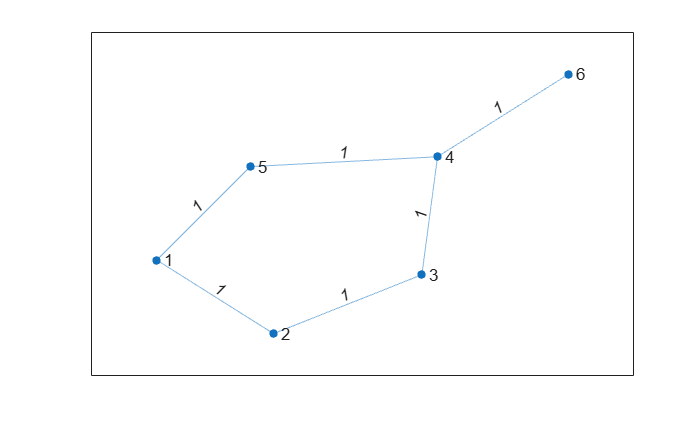Calculate the number of edges in the shortest path between nodes 1 and 6.

`pathLength = shortest_path(A,1,6)`
```pathLength = 3 ```

Open the Test Requirement Set

The `shortest_path_tests_reqs` requirement set contains test requirements that describe the functional behavior that must be tested by a test case. The test requirements are derived from the functional requirements. There are test requirements for the nominal behavior and for the invalid conditions. Open the requirement set in the Requirements Editor.

`testReqs = slreq.open("shortest_path_tests_reqs");`

The class-based MATLAB unit tests in `graph_unit_tests` implement the test cases described in `shortest_path_tests_reqs`. The class contains test methods based on the test requirements from `shortest_path_tests_reqs`. The class also contains the `verify_path_length` method, which the test cases use as a qualification method to verify that the expected and actual results are equal. The class also contains static methods that create adjacency matrices for the test cases.

View the Verification Status

To view the verification status, in the Requirements Editor toolstrip, in the View section, click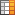Columns and select Verification Status. Three of the functional requirements and one test requirement are missing verification links. The verification status is yellow for each requirement, which indicates that the linked tests have not run.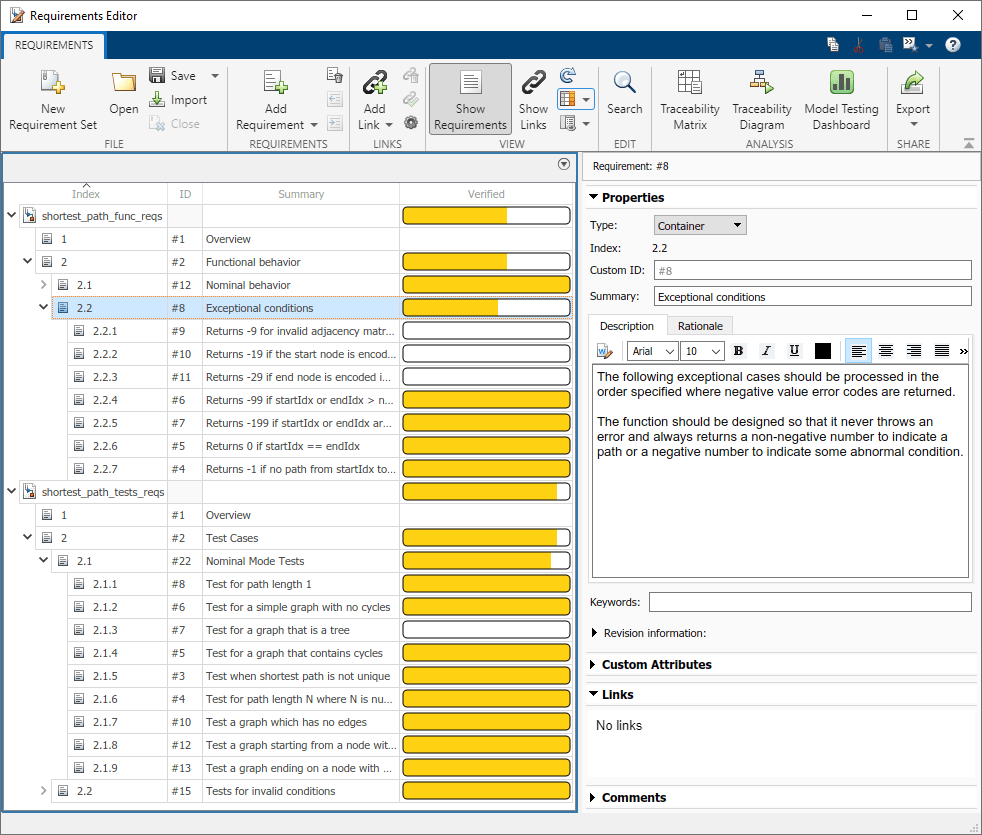Run the tests and update the verification status for the requirement sets by using the `runTests` method.

`status1 = runTests(funcReqs);`
```Running graph_unit_tests .......... .. Done graph_unit_tests __________ ```
`status2 = runTests(testReqs);`
```Running graph_unit_tests .......... ... Done graph_unit_tests __________ ```

The verification status is green to indicate that the linked tests passed. However, some of the requirements do not have links to tests.

Identify Traceability Gaps in the Project

The functional and test requirements are linked to code lines in the `shortest_path` and `graph_unit_tests` files, but the traceability is not complete. Use a traceability matrix to identify requirements that are not linked to tests and to create links to make the requirements fully traceable.

Find the Missing Links with a Traceability Matrix

Create a traceability matrix for both requirement sets with the requirements on the top and the unit tests on the left. For more information about traceability matrices, see Track Requirement Links with a Traceability Matrix

```mtxOpts = slreq.getTraceabilityMatrixOptions; mtxOpts.topArtifacts = {'shortest_path_func_reqs.slreqx','shortest_path_tests_reqs.slreqx'}; mtxOpts.leftArtifacts = {'graph_unit_tests'}; slreq.generateTraceabilityMatrix(mtxOpts)```

In the Filter Panel, in the Top section, filter the matrix to show only the functional requirements not linked to tests by clicking:

• Top > Type > Functional

In the Left section, show only the test functions in the `graph_unit_tests` file by clicking:

• Left > Type > Function

• Left > Attributes > Test

Click Highlight Missing Links in the toolstrip.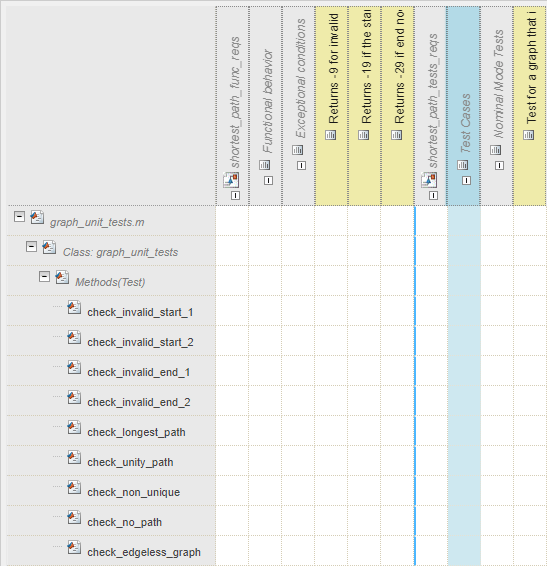The Traceability Matrix window shows the three functional requirements and one test requirement that are missing verification links.

The test requirement 2.1.3, `Test for a graph that is a tree`, is not linked to a test. A tree is a graph in which any two nodes are only connected by one path.

The test case `check_invalid_start_1` tests a tree graph by using the `graph_straight_seq` static method to create the adjacency matrix. Use the` graph_straight_seq` method to view the tree graph.

```A = graph_unit_tests.graph_straight_seq; G = graph(A); plot(G,EdgeLabel=G.Edges.Weight)```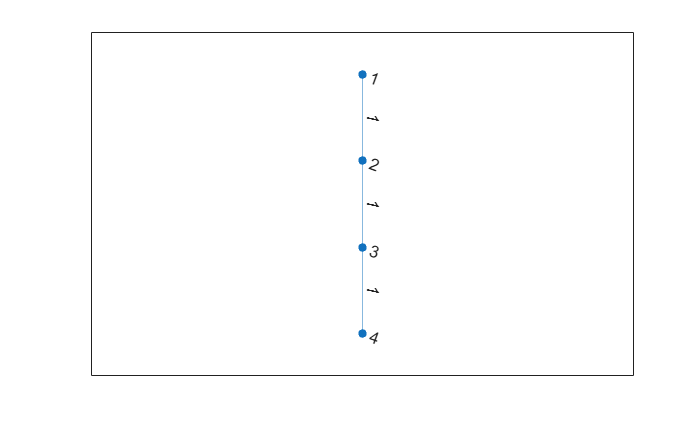Create a link from the `Test for a graph that is a tree` requirement to the `check_invalid_start_1` test case by using the traceability matrix you previously generated.

`slreq.generateTraceabilityMatrix(mtxOpts)`

Click the cell that corresponds to the requirement and the test and select Create. In the Create Link dialog box, click Create.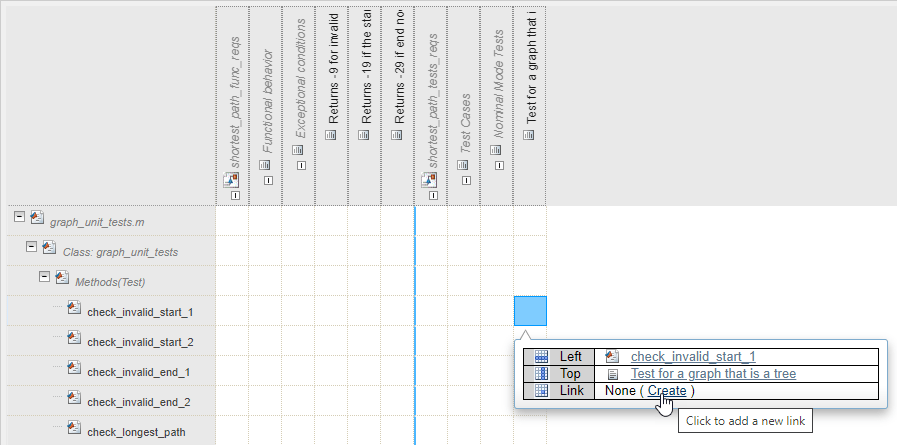Update the verification status in the Requirements Editor by running the tests linked to the test requirements. The `check_invalid_start_1` test verifies the `Test for a graph that is a tree` requirement.

`status3 = runTests(testReqs);`
```Running graph_unit_tests .......... ... Done graph_unit_tests __________ ```

• Requirement 2.2.1: `Returns -9 for invalid adjacency matrices`

• Requirement 2.2.2: `Returns -19 if the start node is encoded incorrectly`

• Requirement 2.2.3: `Returns -29 if end node is encoded incorrectly`

There is a traceability gap for these requirements. You cannot fill this gap by creating links to tests because there are no tests that verify these requirements.

Fix Coverage and Traceability Gaps by Authoring Tests

The three functional requirements that do not have links to tests do have links to lines of code in the `shortest_path` function. Run the tests with coverage to determine if those lines of code in the `shortest_path` function are covered by tests.

Run Tests with Coverage

Use the `RunTestsWithCoverage` script to run the tests with function and statement coverage and view the coverage in a report. For more information, see Generate Code Coverage Report in HTML Format.

`RunTestsWithCoverage`
```Running graph_unit_tests .......... ........ Done graph_unit_tests __________ Code coverage report has been saved to: C:\Users\jdoe\MATLAB\Projects\examples\ShortestPath\coverageReport\index.html ```

Open the coverage report. The error code statements on lines 20, 25, and 30 are not covered by tests.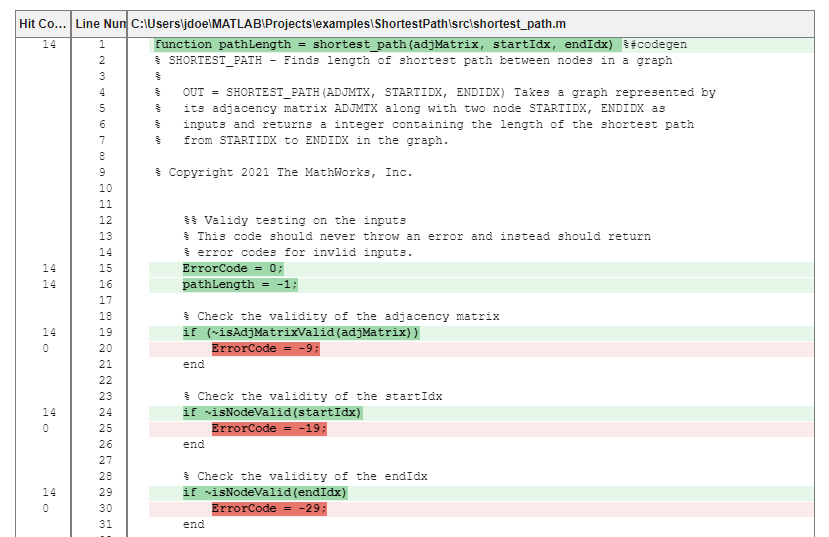Note that the coverage gap for these code lines and the traceability gap for requirements 2.2.1, 2.2.2, and 2.2.3 refer to the same error codes. You can close the coverage and traceability gaps simultaneously by authoring tests for these lines of code and creating links to the requirements.

Improve Coverage by Authoring New Tests

Create tests that improve the coverage for the tests and verify requirements 2.2.1, 2.2.2, and 2.2.2. Open the `graph_unit_tests` test file.

`open("graph_unit_tests.m");`

These functions test the three error codes. Copy and paste the code in line 4, in the test methods section of the `graph_unit_tests` file, then save the file.

```function check_invalid_nonsquare(testCase) adjMatrix = zeros(2,3); startIdx = 1; endIdx = 1; expOut = -9; verify_path_length(testCase, adjMatrix, startIdx, endIdx, expOut, ... 'Graph is not square'); end function check_invalid_entry(testCase) adjMatrix = 2*ones(4,4); startIdx = 1; endIdx = 1; expOut = -9; verify_path_length(testCase, adjMatrix, startIdx, endIdx, expOut, ... 'Adjacency matrix is not valid'); end function check_invalid_noninteger_startnode(testCase) adjMatrix = zeros(4,4); startIdx = 1.2; endIdx = 1; expOut = -19; verify_path_length(testCase, adjMatrix, startIdx, endIdx, expOut, ... 'Start node is not an integer'); end function check_invalid_noninteger_endnode(testCase) adjMatrix = zeros(4,4); startIdx = 1; endIdx = 2.2; expOut = -29; verify_path_length(testCase, adjMatrix, startIdx, endIdx, expOut, ... 'End node is not an integer'); end ```

Rerun the tests with coverage and open the coverage report.

`RunTestsWithCoverage`
```Running graph_unit_tests .......... ........ Done graph_unit_tests __________ Code coverage report has been saved to: C:\Users\jdoe\MATLAB\Projects\examples\ShortestPath\coverageReport\index.html ```

The tests now cover the error code statements.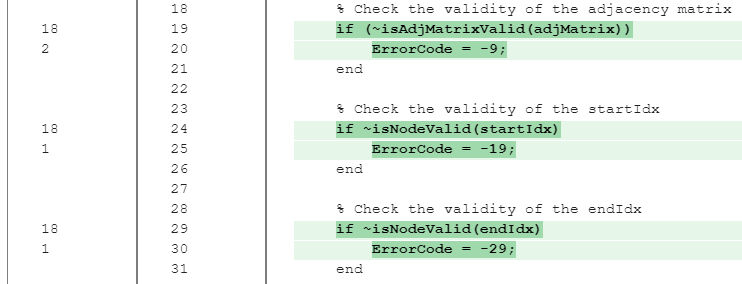However, there is a statement on line 97 that the tests do not cover. The conditions that require the tests to cover the statement on line 97 also cause the `return` on line 87 to execute, which means that the statement on 97 is not reachable and is dead logic.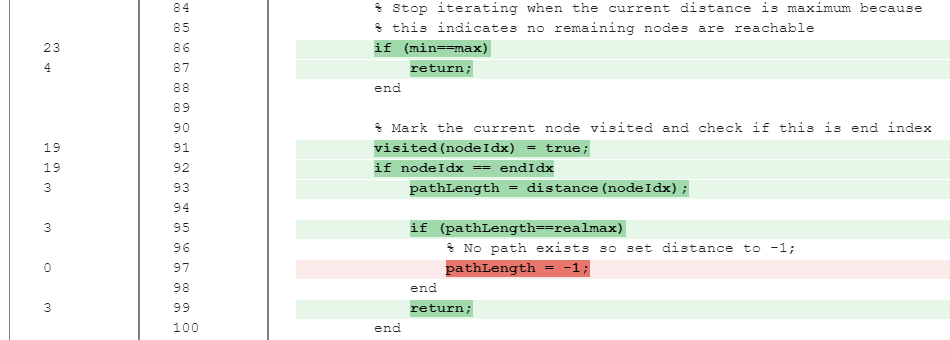Fix Requirement Traceability Gaps

Regenerate the traceability matrix, apply the same filters from before, then click Highlight Missing Links in the toolstrip.

`slreq.generateTraceabilityMatrix(mtxOpts)`

• Top > Type > Functional

• Left > Type > Function

• Left > Attributes > Test

Create links between the error code requirements and the new tests.Update the verification status in the Requirements Editor by re-running the tests linked to both requirement sets.

`status4 = runTests(funcReqs);`
```Running graph_unit_tests .......... .. Done graph_unit_tests __________ ```
`status5 = runTests(testReqs);`
```Running graph_unit_tests .......... ... Done graph_unit_tests __________ ```

All requirements have links to tests and all tests pass.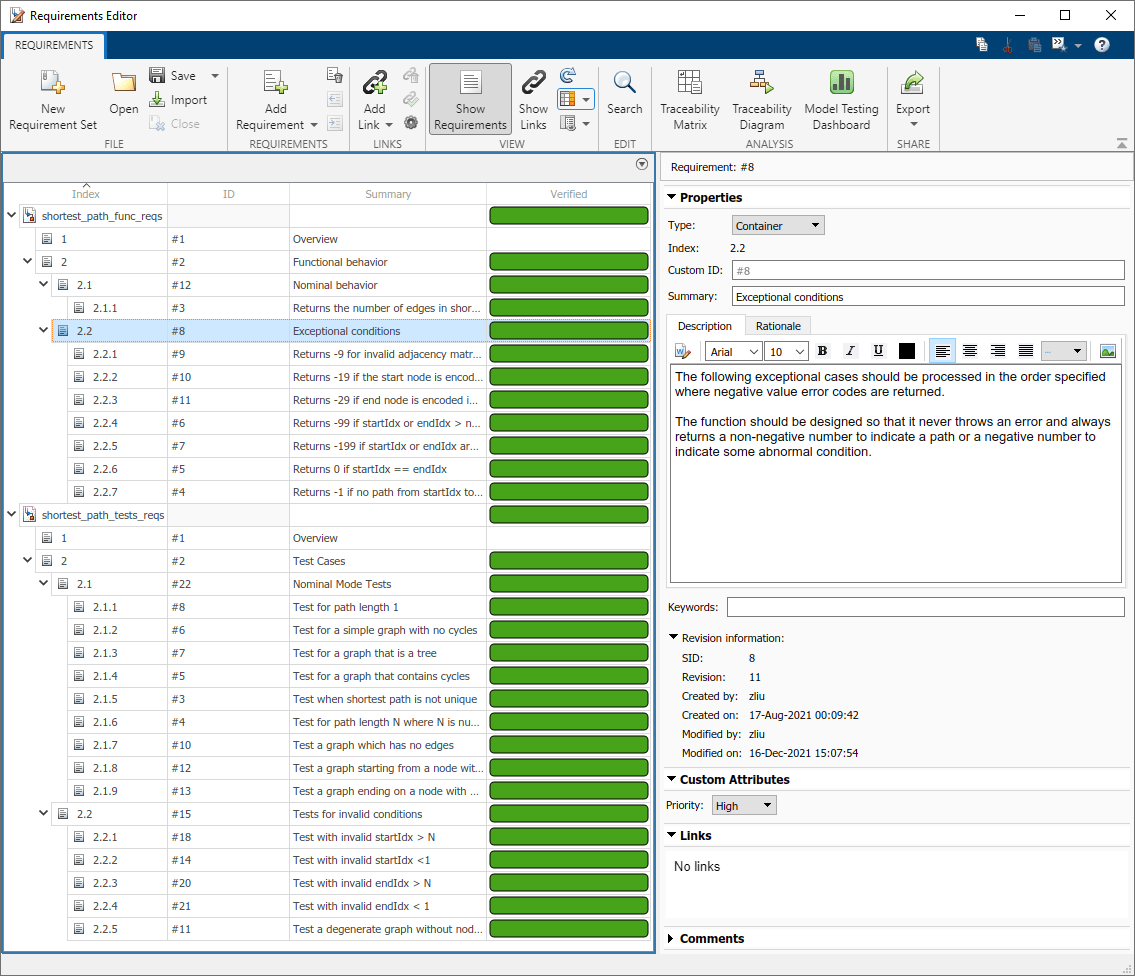Generate and Verify Code from the Algorithm

You can generate code from the `shortest_path` algorithm by using MATLAB® Coder™. Use `coder.typeof` to define a variable-sized double array with a maximum size of 100x100, and a scalar double to use as inputs in the generated MEX function.

```mtxType = coder.typeof(ones(100,100),[],1); scalarDblType = coder.typeof(1);```

Generate a MEX function from the `shortest_path` algorithm with the specified input types.

`codegen shortest_path -args {mtxType, scalarDblType, scalarDblType}`
```Code generation successful. ```

Use `coder.runTest` to rerun the tests from the `graph_unit_tests` file by executing the MEX file instead of the `shortest_path` function.

`coder.runTest("graph_unit_tests","shortest_path")`
```Running graph_unit_tests .......... ........ Done graph_unit_tests __________ 1×18 TestResult array with properties: Name Passed Failed Incomplete Duration Details Totals: 18 Passed, 0 Failed, 0 Incomplete. 0.06382 seconds testing time. Name Passed Failed Incomplete Duration Details _______________________________________________________ ______ ______ __________ _________ ____________ {'graph_unit_tests/check_invalid_nonsquare' } true false false 0.011649 {1×1 struct} {'graph_unit_tests/check_invalid_entry' } true false false 0.0024322 {1×1 struct} {'graph_unit_tests/check_invalid_noninteger_startnode'} true false false 0.0018891 {1×1 struct} {'graph_unit_tests/check_invalid_noninteger_endnode' } true false false 0.0025271 {1×1 struct} {'graph_unit_tests/check_invalid_start_1' } true false false 0.0054457 {1×1 struct} {'graph_unit_tests/check_invalid_start_2' } true false false 0.004266 {1×1 struct} {'graph_unit_tests/check_invalid_end_1' } true false false 0.0027437 {1×1 struct} {'graph_unit_tests/check_invalid_end_2' } true false false 0.0035073 {1×1 struct} {'graph_unit_tests/check_longest_path' } true false false 0.0031678 {1×1 struct} {'graph_unit_tests/check_unity_path' } true false false 0.0026999 {1×1 struct} {'graph_unit_tests/check_non_unique' } true false false 0.0028623 {1×1 struct} {'graph_unit_tests/check_no_path' } true false false 0.0029491 {1×1 struct} {'graph_unit_tests/check_edgeless_graph' } true false false 0.0025469 {1×1 struct} {'graph_unit_tests/check_edgeless_start' } true false false 0.003268 {1×1 struct} {'graph_unit_tests/check_edgeless_end' } true false false 0.0029977 {1×1 struct} {'graph_unit_tests/check_edgeless_graph_self_loop' } true false false 0.0024533 {1×1 struct} {'graph_unit_tests/check_start_end_same' } true false false 0.0036799 {1×1 struct} {'graph_unit_tests/check_invalid_idx_empty_adj' } true false false 0.0027352 {1×1 struct} ```

The tests pass when they execute the generated MEX function. The tests verify the generated code.

## Version History

Introduced in R2022a